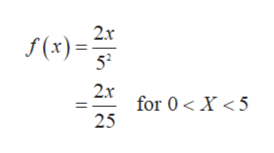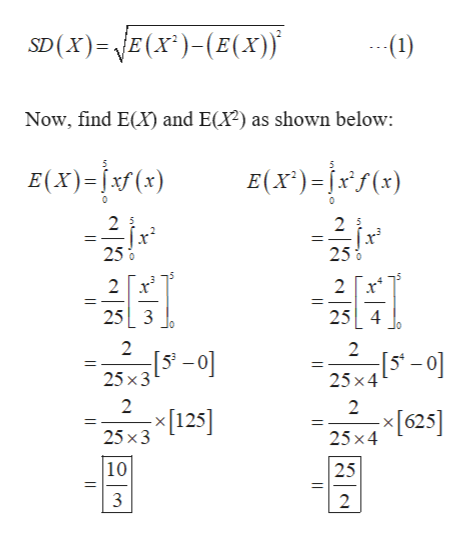Suppose that the probability density function of the length of computer cables is f (x) = 2x/(52) for x between 0 and 5 meters. Determine the standard deviation of the cable length. Please enter the answer to 2 decimal places.

Question

Suppose that the probability density function of the length of computer cables is f (x) = 2x/(52) for x between 0 and 5 meters. Determine the standard deviation of the cable length. Please enter the answer to 2 decimal places.

Step 1

Let X be the continuous random variable which denotes the length of the computer cables.

The probability density function of X is given by,help_outlineImage Transcriptionclose2x f(x) 52 2x for 0X <5 25 fullscreen
Step 2

The standard deviation of X is ca...help_outlineImage TranscriptioncloseSD(x) E(x)-(E(x) (1) Now, find E(X) and E(X2) as shown below: E(X)-() E(x)=x) 0 2 2 2 5 25% 250 0 2 2 25 3 25 4 2 2 25x3 25x4 2 2  25 x3 x625] 25x4 10 25 2 |I fullscreen

Want to see the full answer?

See Solution

Want to see this answer and more?

Our solutions are written by experts, many with advanced degrees, and available 24/7

See Solution
Tagged in

Other